maths > statistics-basics

Tally and Table Representation of Data

what you'll learn...

overview

In this page, the tally and table representations are introduced with example

•  Tally representation uses vertical bars (that are grouped into $5$$5$) is used to collect data.

•  In table representation, data is given in tabular form.

on-going data collection

In a plate, oranges, apples, bananas, and mangoes were available. A group of students took one fruit each as given in the data.

The data is given as

$2$$2$ students took oranges
$2$$2$ students took apples
$5$$5$ students took mangoes
$1$$1$ student took banana

Continuing on, the next student takes an orange, and then the following student takes a mango.

To update the information, the $2$$2$ oranges is changed to $3$$3$; and $5$$5$ mangoes is changed to $6$$6$.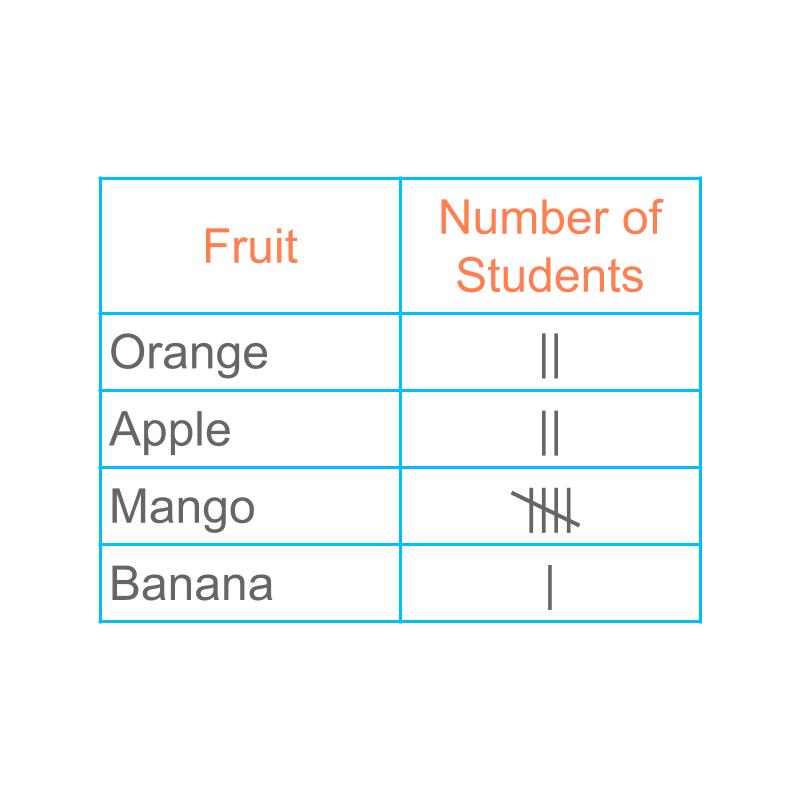To continue on an on-going data collection, the "tally" representation helps.

The data is given in tally representation in the figure. Note the number of vertical lines given in each row. The data value $2$$2$ is represented with $2$$2$ vertical lines. A collection of $5$$5$ is represented with a diagonal line on four vertical lines.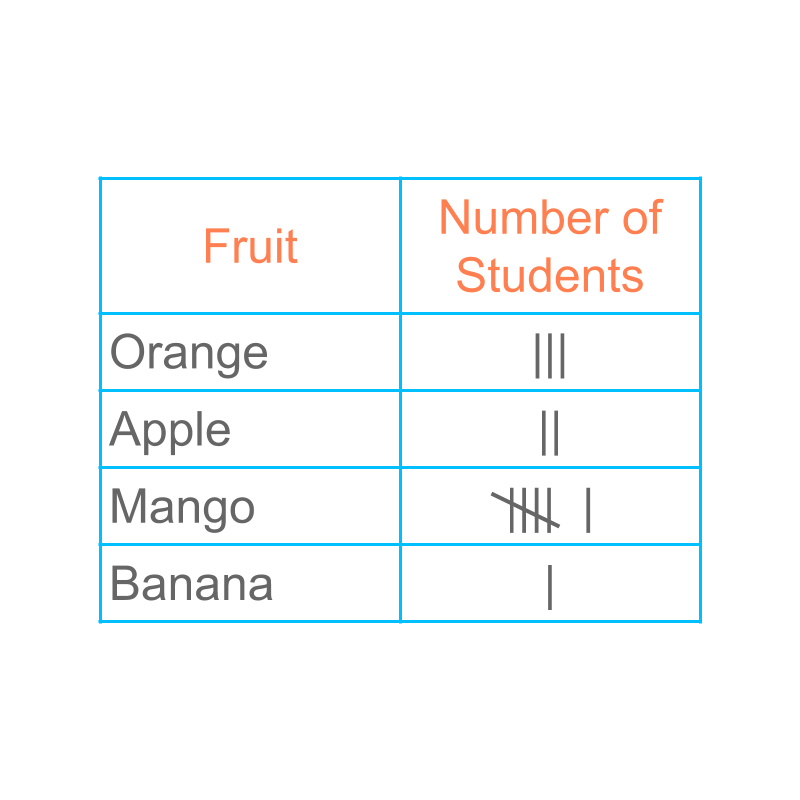Continuing on, the next student takes an orange, and then the following student takes a mango. The tally representation is updated easily.

The word "tally" means: current amount; ongoing count.

represent, once completed

Consider the tally representation of data given in the figure.

It is not straight forward to find the number of students who took mangoes. Some counting and adding has to be done to find the answer.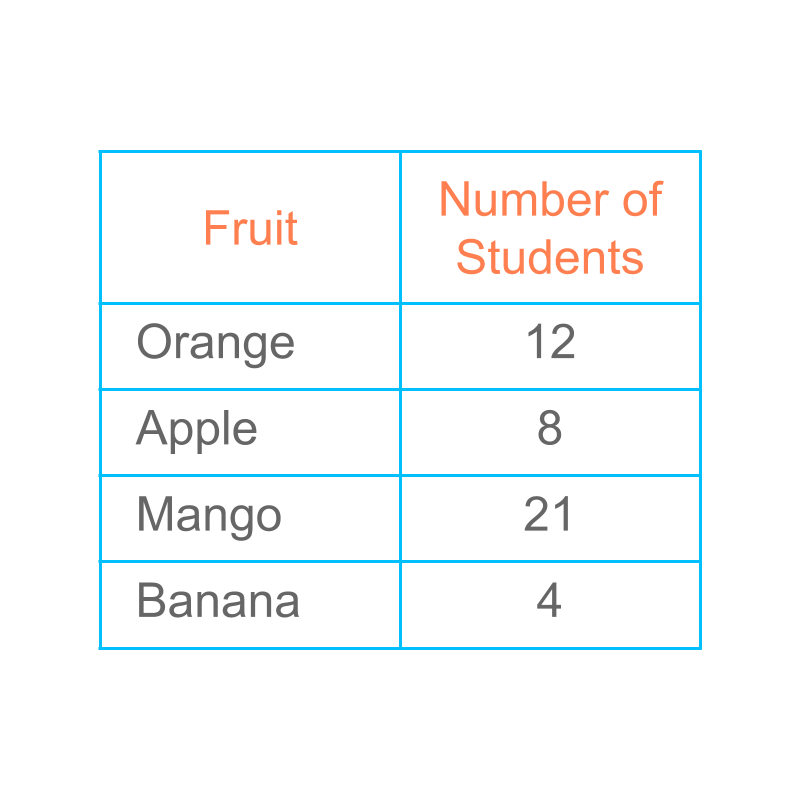Tally representation is suitable to record an on-going data collection. Once the data collection is completed, the same can be given in the tabular form.

The data given in tally is equivalently given in a "table". This representation is also called "tabulation of data".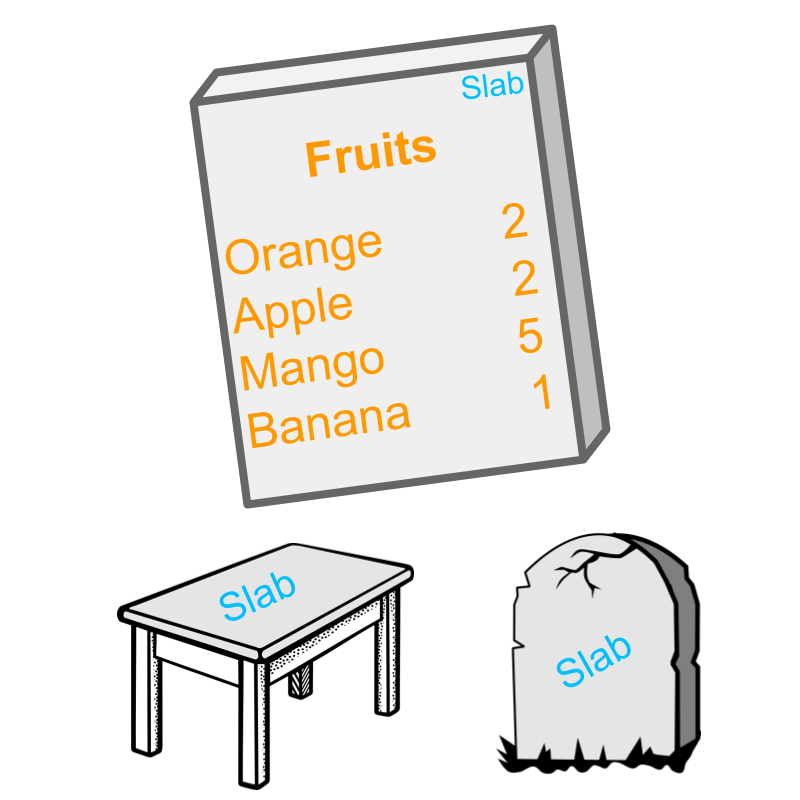The word "table" means: a flat rectangular surface. The surface can be vertical with inscriptions.

summary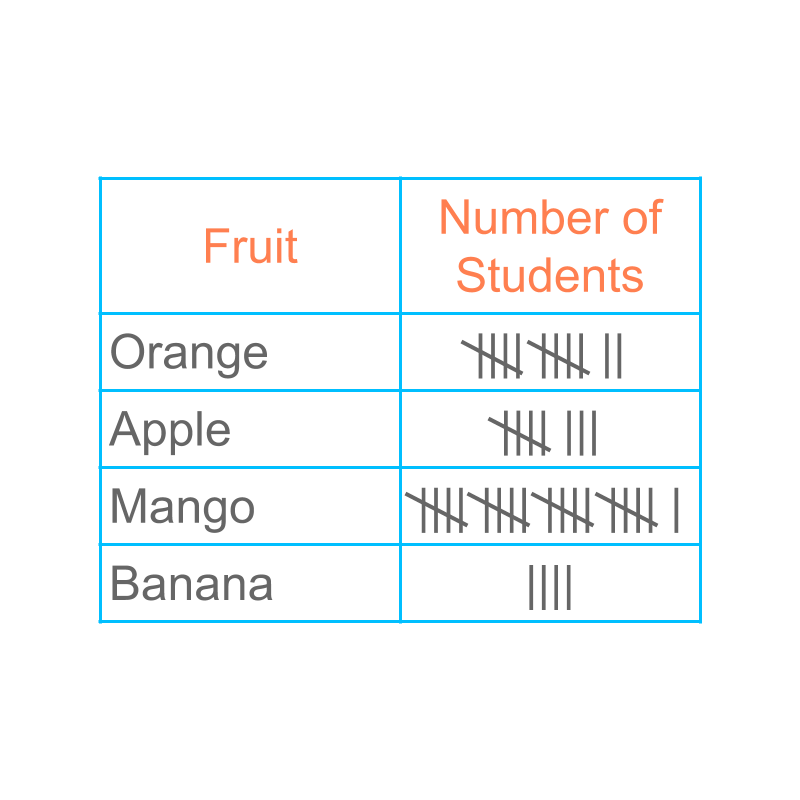Presenting Data with Tally Marks : Vertical bars (that are grouped into $5$$5$) is used to collect data.Presenting Data in a Table : The data is given in a tabular form.

Outline